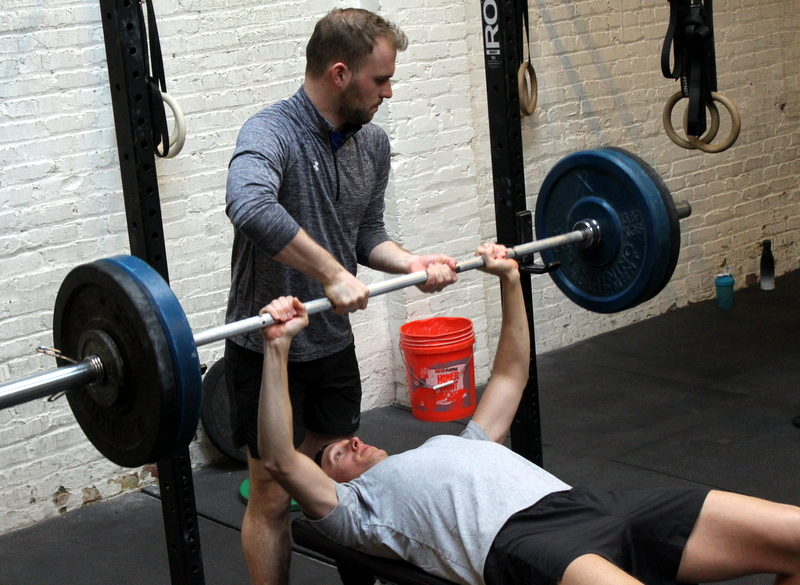## Friday, March, 29, 2019

### Friday, March, 29, 2019as many rounds as possible in 20 minutes:
15x strict pull-up
30x pushup
45x squat
400m run

### 1 Comment

1.–Scores–
Anna G.: 2 rounds + 75m (sub: 2/3x band, 2/3x incline pushup)
Borja G.: 2 rounds + 7x squat
Kevin K.: 2 rounds + 45x squat (sub: 1/2x strict)
Matt H.: 2 rounds + 200m (sub: 20x sit-up for pull-up)
Zach F.: 3 rounds + 13x pushup (sub: 1/2x strict)
Aaron B.: 2 rounds + 75m (sub: 2/3x band, 2/3x pushup)
Al C.: 3 rounds + 9x strict
Tommy W.: 3 rounds + 8x strict (sub: 1/2x strict, 2/3x pushup)
Walt C.: 3 rounds + 4x strict (sub: 2/3x strict)
David C.: 3 rounds + 7x strict (sub: 2/3x strict, 2/3x pushup)
Chase H.: 3 rounds + 30x squat
Zach D.: 3 rounds + 28x pushup
Chris C.: 3 rounds + 22x pushup
Jason S.: 3 rounds + 19x squat (sub: 1/2x strict, 12-cal bike)
Adrian P.: 2 rounds + 375m (sub: 1/2x strict, 2/3x pushup)
Alissa G.: 3 rounds + 1x strict (sub: no run)
Will O.: 3 rounds + 5x pushup (sub: 2/3x strict)
Alli P.: 3 rounds + 7x strict (sub: 2/3x strict)
Dave R.: 2 rounds + 350m
Hari P.: 2 rounds + 300m
Andrew B.: 2 rounds + 380m (sub: 2/3x band, 2/3x pushup)
Diana G.: 2 rounds + 350m (sub: strict bodyrow, sit-up)
Carla J.: 2 rounds + 6x squat (sub: 2/3x band)
Peter Mc.: 3 rounds
Cristian D.: 2 rounds + 30x squat (sub: 2/3x band, 2/3x pushup)
Diana D.: 2 rounds + 41x squat (sub: 2/3x band, 2/3x pushup)
Kate G.: 3 rounds + 1x pushup (sub: 2/3x band, 2/3x pushup)
Mead O.: 3 rounds (sub: 2/3x strict bodyrow, 2/3x knee pushup)
Chenelyn B.: 2 rounds + 45x squat (sub: 2/3x strict bodyrow, 2/3x knee pushup)
Chris H.: 3 rounds + 31x squat
Dave K.: 3 rounds + 5x strict (sub: 2/3x strict, 2/3x pushup)
Ralph A.: 2 rounds + 10x pushup (sub: 2/3x band, 2/3x pushup)
Francisco L.: 2 rounds + 250m
Charlie S.: 2 rounds + 200m
Mel F.: 2 rounds + 16x pushup
Ryan A.: 3 rounds (sub: 2/3x band, 2/3x pushup)
Lisa C.: 2 rounds + 275m (sub: 2/3x band, 2/3x pushup)
Joya TD.: 2 rounds + 300m (sub: 2/3x strict, 2/3x pushup)
Simon B.: 2 rounds + 19x pushup (sub: 2/3x band, 2/3x pushup)
Michael H.: 2 rounds + 23 double unders (sub: 75x double under instead of 400m run)
Gabriel F.: 1 round + 300m run
Carl L.: 2 rounds + 200m run
Matthew R.: 2 rounds + 10 pull-up (sub: 2/3x band)
Katie G.: 2 rounds + 5 pull-up (sub: 3 strict then up to 2/3x band, 2/3x pushup)
Joe T.: 3 rounds +13 pull-up
Seth C.: 2 rounds +11 push-up
Seth B.: 2 rounds + 10 push-up (sub: 2/3x band)
Mark Co.: 2 rounds + 20 air squats
Christopher P.: 2 rounds + 300m run
Ross B.: 3 rounds (sub: green band)
Josh M.: 3 rounds + 26 push-up
Bill G.: 3 rounds + 100m run (lunges)
Charles B.: 3 round + 5 push-up
Collin S.: 2 rounds + 100m run
Charlie K.: 2 rounds + 25 push-up
Mark Ab.: 3 rounds + 31 squats (sub: DL @ 225-lbs., 30 hollow rocks)
RJ A.: 2 rounds + 10 pull-up (sub: 2/3x pull-up)
Daniel C.: 2 rounds +24 push-up (sub: 2/3x pull-up)
Dan D.: 2 rounds + 10 pull-up
Beth K.: 2 rounds + 21 air squats (sub: 4 strict then up to 2/3x band, 2/3x pushup/knees, parallel squat)
Max M.: ???